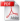Kamakura, Japan

## Exact Solution for Two-Phase Flow in Porous Medium with a Material Discontinuity [testing]

This is the online implementation of the 1D integral solution of two-phase flow in porous media with a material discontinuity Fučík et al. (2008). Please, cite our work (see references below).

# Parameters

If you see this, please, leave this form field blank and invest in CSS support.

Model selection

Model selection

 Fluid properties $\mu_w$ [kg/m/s] dynamic viscosity of the wetting phase $\mu_n$ [kg/m/s] dynamic viscosity of the non-wetting phase Material properties Left domain $x<0$ Right domain $x>0$ $\phi$  porosity $K$ [m$^2$] intrinsic permeability $S_{wr}$  residual saturation of the wetting phase $S_{nr}$  residual saturation of the non-wetting phase $S_i$  initial saturation Brooks and Corey model parameters Left domain $x<0$ Right domain $x>0$ $p_d$ [Pa] Brooks and Corey model parameter: entry pressure $\lambda$  Brooks and Corey model parameter: pore size distribution index van Genuchten mode parameters Left domain $x<0$ Right domain $x>0$ $\alpha$ [1/Pa] van Genuchten model parameter $m$  van Genuchten model parameter $n$  van Genuchten model parameter Problem parameters $R$  flux parameter $t$ [s] the solution will be plotted and exported at this time Computation parameters nodes length of the discrete vector max_iter maximum number of iterations $\epsilon$ stopping criterion of the iterations

# References

•pdf R.Fučík, J. Mikyška, T. H. Illangasekare and M. Beneš: Semianalytical Solution for Two-Phase flow in Porous Media with a Discontinuity Vadose Zone Journal 2008 vol. 7, no. 3: pages 1001-1009
•pdf R.Fučík, J. Mikyška, T. H. Illangasekare and M. Beneš: An Improved Semi-Analytical Solution for Verification of Numerical Models of Two-Phase Flow in Porous Media Vadose Zone Journal 2007 no. 6: pages 93-104pdf (1,01 MB) corrected version